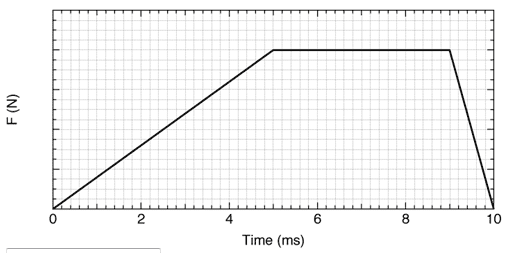# What is the maximum value of the contact force during the collision?

## Homework Statement

The Figure shows an approximate representation of the contact force versus time during the collision of a 42-g tennis ball with a wall. The initial velocity of the ball is 35 m/s perpendicular to the wall; it rebounds with the same speed, also perpendicular to the wall. What is the maximum value of the contact force during the collision?P = mv

## The Attempt at a Solution

I know P = 35 (42/1000) = 1.47 N*m

What is next step? Thank you!

Last edited:

## Answers and Replies

thrill3rnit3
Gold Member
You're trying to find the value of the maximum force, which is the value where the graph flattens out.

Solve for J, using the same equation

Po + J = Pf

J is the area of the figure.

Think about it for a few minutes and post again if you still need more help. I can't just spoonfeed you with the answer :tongue:

but in the problem, there are no number is given on vertical axis!!!

thrill3rnit3
Gold Member
but in the problem, there are no number is given on vertical axis!!!

That's what the problem asks you to figure outso the 1st Area is 0.5 * 0.005 * F

2nd Area is 0.5 * 0.004 * F

3rd Area is 0.5 * 0.001 * F

So I add all them up: 0.005F, then 1.47 - 0.005F = (42/1000)vf

now I have 2 unknown ???

Last edited:
thrill3rnit3
Gold Member
so the 1st Area is 0.5 * 0.005 * F

2nd Area is 0.5 * 0.004 * F

3rd Area is 0.5 * 0.001 * F

So I add all them up: 0.005F, then 1.47 - 0.005F = (42/1000)vf

now I have 2 unknown ???

You did a good job with the 0.005F. What does 0.005F equal? (Hint: read the previous posts)

Last edited:
0.005 F = mv right?

thrill3rnit3
Gold Member
Hint #2: It's in my first response0.005 F = Pf - P(o) = > 0.005 F = m [vf - v(o)], right?

Andrew Mason
Science Advisor
Homework Helper

## Homework Statement

The Figure shows an approximate representation of the contact force versus time during the collision of a 42-g tennis ball with a wall. The initial velocity of the ball is 35 m/s perpendicular to the wall; it rebounds with the same speed, also perpendicular to the wall. What is the maximum value of the contact force during the collision?P = mv

## The Attempt at a Solution

I know P = 35 (42/1000) = 1.47 N*m

What is next step? Thank you!
The maximum force is easy to locate on the graph but the problem is we do not know how the vertical axis is calibrated - ie how many Newtons per square. You have to work that out from the information given.

What is the total change in momentum? How is that related to the total impulse? What aspect of the graph represents the total impulse? How does that enable you to determine the vertical scale of the graph?

AM

but 0.005 F = (42/1000) * 35 => F = 294 N => Wrong!!!

thrill3rnit3
Gold Member
0.005 F = Pf - P(o) = > 0.005 F = m [vf - v(o)], right?

This looks right. I deleted my other post because I quoted the wrong response.

Remember that 0.005F would be negative in this case because it's a force opposite the motion.

but what is vf ???

thrill3rnit3
Gold Member
it rebounds with the same speed

quoted directly from the problem

Oh! I see!!! So -0.005F = -2 v m => F = 588 N ???

thrill3rnit3
Gold Member
Oh! I see!!! So -0.005F = -2 v m => F = 588 N ???

Yep, that's the answer I have.

588 N = incorrect :(

thrill3rnit3
Gold Member
I went back to your calculation of your area:

2nd Area is 0.5 * 0.004 * F

That 0.5 shouldn't be there.

thrill beat me to it..

-5*10^-9 F = -2 m v ????

thrill3rnit3
Gold Member
-5*10^-9 F = -2 m v ????

...no.

The area calculation is correct except for the tiny mistake I pointed out.

GOT IT! Thanks for your patience again! ;)

thrill3rnit3
Gold Member
I'm sorry I didn't catch that mistake a few posts back. Today was just a tiring day for me, in fact I'm about to go to bed now :rofl:

Again I'm glad it worked out well for youGood night! See u tomorrow LOL

thrill3rnit3
Gold Member
Good night! See u tomorrow LOL

More problems?? :rofl:

Just learn how to manipulate the equation based on the given info and what you're looking for. Most of these problems are similar in nature and ask about one specific thing or another.